# Class 10 Board Exams - Top Maths Formulae You Should Know

###### ByRamraj Saini###### Synopsis

Maths can often be a source of anxiety for students, especially during exams. Even candidates who are otherwise well-prepared and have grasped concepts may falter when faced with a question paper. Knowing which topics carry the maximum weight from the exam perspective can help boost confidence in the final stretch of pre-exam preparation. Here’s a list of formulae from Class 10 Maths that carry the most marks in the board exam.###### Synopsis

Maths can often be a source of anxiety for students, especially during exams. Even candidates who are otherwise well-prepared and have grasped concepts may falter when faced with a question paper. Knowing which topics carry the maximum weight from the exam perspective can help boost confidence in the final stretch of pre-exam preparation. Here’s a list of formulae from Class 10 Maths that carry the most marks in the board exam.

While every component of the Mathematics curriculum is important for building an interest in and understanding of the discipline as a whole, they don’t all carry equal weight in board exams. For the final revision for the Central Board of Secondary Education (CBSE) Class 10 Maths exam, knowing which ones carry the most marks and the most critical formulae associated with them can help build confidence.

The NCERT Class 10 Mathematics textbook contains the following units

• Algebra

• Geometry

• Trigonometry

• Statistics and Probability

• Mensuration

• Coordinate Geometry

• Number Systems

Given below is a list of the most important Maths formulae for CBSE Class 10 board exams, in the decreasing order of the marks they carry.

## Algebra: 20 Marks

This unit contains the maximum marks in the Class 10 CBSE Maths board exam and includes chapters like Polynomials, Pair of Linear Equations in two Variables, Quadratic Equations, and Arithmetic Progression. Here are the important formulae for the Class 10 board exam from each chapter.

### Polynomials

This chapter mainly discusses the definition of a polynomial, degree of a polynomial, the zeros of a polynomial, the fundamental theorem of algebra, the division algorithm for polynomials, synthetic division, and the zeros theorem.

These are the most important formulae:

(a+b)2 = a2+2ab+b2

(a-b)2 = a2-2ab+b2

(a+b)(a-b) = a2-b2

(x+a)(x+b)=x2+(a+b)x+ab

a3-b3=(a-b)(a2+ab+b2)

a3+b3=(a+b)(a2-ab+b2)

(a+b)3=a3+b3+3ab(a+b)

(a-b)3=a3-3ab(a-b)+b3

(a+b+c)2=a2+b2+c2+2ab+2bc+2ca

If a quadratic equation is given as ax2+bx+c=0 then

Sum of zeros = α+β = -b/a=-(coefficient of x)/coefficient of x2

Product of zeros =αβ = c/a=-(coefficient of x)/coefficient of x2

If a cubic equation is given as ax3+bx2+cx+d=0 then

Sum of zeros = α+β+γ = -b/a=-(coefficient of x2)/coefficient of x3

Sum of multiple of alternate zeros = αβ+βγ+γα =c/a = coefficient of x/coefficient of x3

Product of zeros =αβγ= -d/a=-(constant term)/coefficient of x3

If p(x) and g(x) are two polynomials such that g(x) is not equal to 0, then we can find polynomials q(x) and r(x) such that the following equation holds:

p(x) = g(x) * q(x) + r(x)

### Pair Of Linear Equations In Two Variables

There are three possible ways to solve a pair of linear equations in two variables: graphing, substitution method, and elimination method.

• The graphing method involves plotting the two equations on the same coordinate plane and finding the point(s) of intersection.

• The substitution method involves solving one of the equations for one variable in terms of the other and substituting this expression into the other equation to solve for the remaining variable.

• The elimination method involves adding or subtracting the two equations to eliminate one of the variables.

If a pair of linear equations in two variables has exactly one solution, it is called a consistent pair of equations. If it has no solutions, it is called an inconsistent pair. If it has infinite solutions, it is called a dependent pair. The following are important formulae from this chapter.

If a pair of linear equations is given as

a1x+b1y+c1 =0

a2x+b2y+c2=0

 Comparison Of Ratios Graphical Interpretation Algebraic Interpretation (a1/a2) ≠ (b1/b2) Intersecting lines Exactly one or unique solution a1/a2=b1/b2=c1/c2 Coincident lines Infinitely many solutions a1/a2=b1/b2≠c1/c2 Parallel lines No solutions

When there is a unique solution then

x/(b1c2-b2c1)=y/(c1a2-c2a1)=1/(a1b2-a2b1)

This formula can be remembered as the diagram given below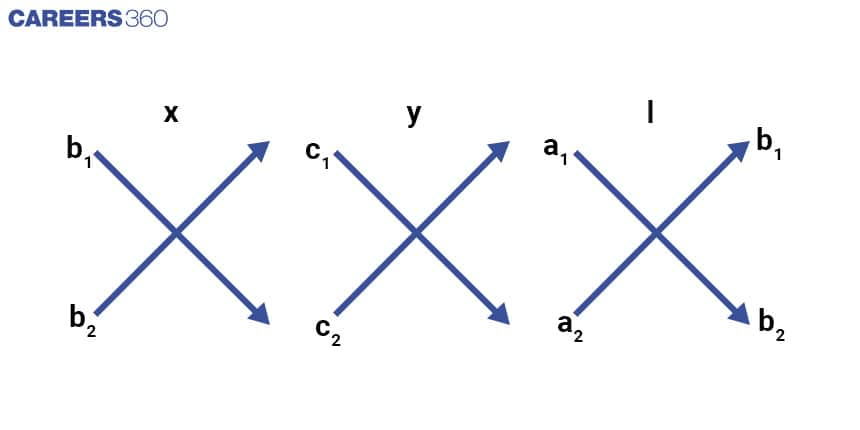This chapter contains concepts of quadratic equations, solution of quadratic equation with factorisation method, completing the square method, and lastly the nature of roots.

The standard form of a quadratic equation is ax2 + bx + c = 0, where a, b, and c are constants and x is the variable. The solutions to a quadratic equation are also known as the roots of the equation and can be found using the quadratic formula:

x ={-b±√(b2-4ac)}/2a

If the value of the discriminant b2-4ac is positive, the equation has two distinct real roots. If the value of the discriminant is zero, the equation has one repeated real root. If the value of the discriminant is negative, the equation has no real roots.

### Arithmetic Progression

In an arithmetic progression (AP), the difference between consecutive terms is always constant. Some important formulae for arithmetic progressions are:

If an AP is given as a1,a2,a3,..................an

Common difference = d=a2-a1=a3-a2=a4-a3=an-an-1

Nth term of AP = Tn=a+(n-1)d

Sum of n term of AP = Sn=(n/2)[2a+(n-1)d]=(n/2)[T1+Tn]

## Geometry: 15 Marks

This unit contains three chapters – Triangles, Circles, and Constructions. Below, students can find important formulae from each chapter.

### Triangles

This chapter contains concepts of similar triangles, criteria of similarity of triangles, calculation of area of similar triangles, and Pythagoras’ theorem. Here are some important formulae from this chapter.

• If a line is drawn parallel to one side of a triangle and intersects the other two sides at two different points, the lengths of the segments into which the other two sides are divided will be in the same ratio and vice versa.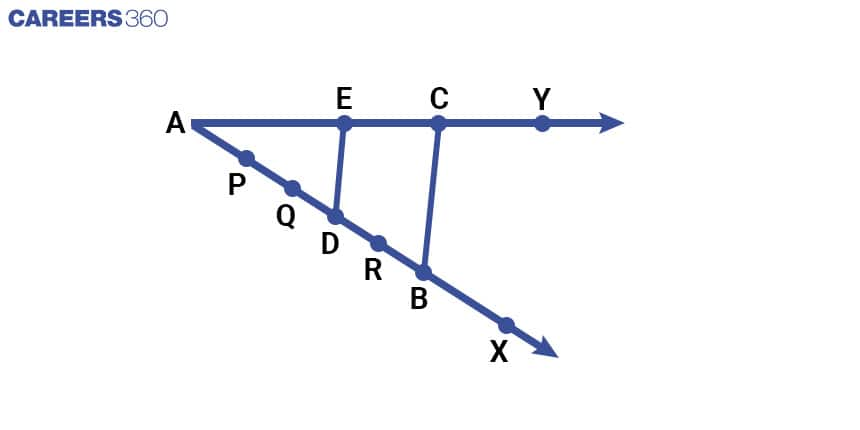• Similarity Of Triangles

1. AA (Angle-Angle) Similarity: If two triangles have two pairs of corresponding angles that are congruent (equal in measure), then the triangles are similar.

2. SAS (Side-Angle-Side) Similarity: If two triangles have two pairs of corresponding sides that are in the same ratio, and the included angles are congruent, then the triangles are similar.

3. SSS (Side-Side-Side) Similarity: If two triangles have three pairs of corresponding sides that are in the same ratio, then the triangles are similar.

4. HL (Hypotenuse-Leg) Similarity: If two right triangles have one pair of corresponding legs that are in the same ratio, and the hypotenuses of the triangles are in the same ratio, then the triangles are similar.

• The ratio of the areas of two similar triangles is equal to the ratio of the squares of their corresponding sides.

ar(ABC)/ar(PQR)=(AB/PQ)2=(BC/QR)2=(CA/RP)2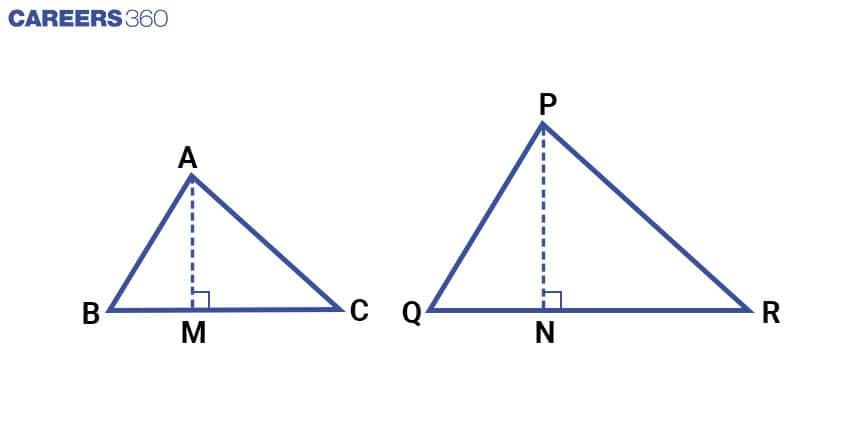• In a right triangle, the length of the hypotenuse is equal to the square root of the sum of the squares of the lengths of the other two sides.

AC2=AB2+BC2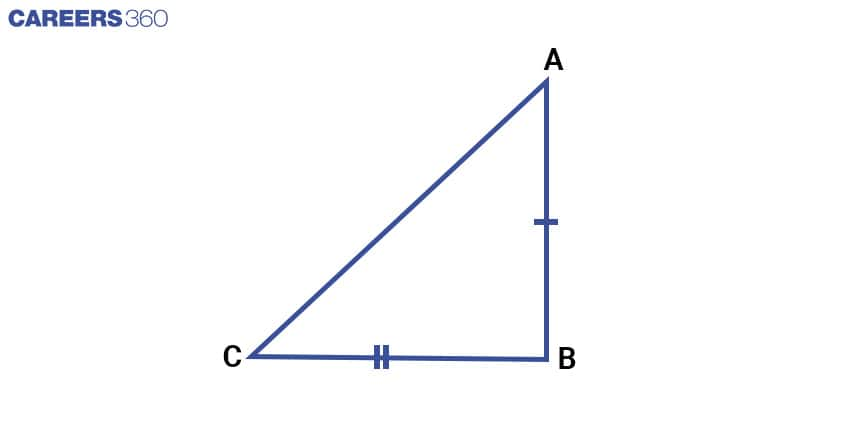### Circles

This chapter describes concepts of tangent and tangent to a circle. Following are some important concepts from this chapter.

• At any point of contact, the tangent to a circle is perpendicular to the radius drawn from the point of contact.

• It is not possible to draw a tangent from a point inside a circle to the circle.

• A single tangent can be drawn from a point on the circle to the circle.

• Two tangents can be drawn from a point outside the circle to the circle.

• The lengths of tangents drawn from an external point to a circle are equal.

### Constructions

This chapter discusses concepts of division of a line segment and construction of a tangent to a circle. Particularly, it contains the concepts of dividing a line segment in a given ratio, constructing a triangle similar to a given triangle as per the given scale factor, and constructing the tangents to a circle from a point outside it.

## Trigonometry: 12 Marks

This unit includes two chapters Introduction to Trigonometry and Some Applications of Trigonometry. Here students can find important formulae for the board examination from each chapter.

### Trigonometry And Its Applications

In A right angle Δ PQR, the ratios are defined as: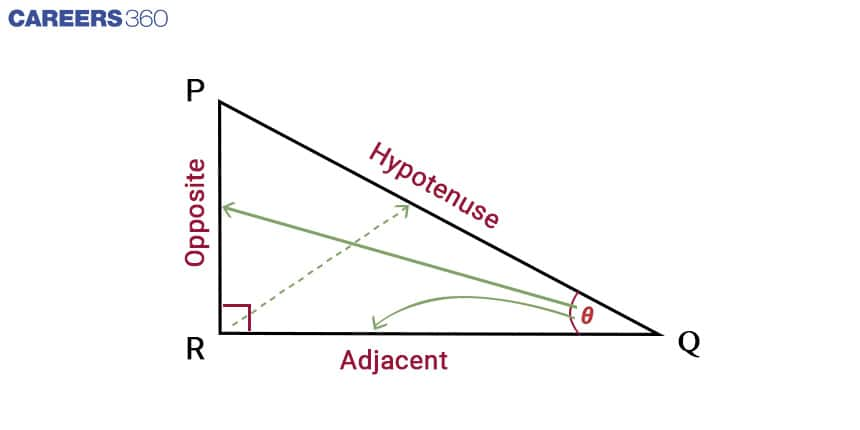sin Q = (opposite side to ∠Q)/(hypotenuse) = PR/PQ

cos Q = (adjacent side to ∠Q)/(hypotenuse) = RQ/PQ

tan Q = (opposite side to ∠Q)/(adjacent side to ∠Q) = PR/RQ

= sin ∠Q/cos ∠Q

cosec Q= 1/sin Q = (hypotenuse)/ (side opposite to ∠Q) = PQ/PR

sec Q = 1/cos Q = (hypotenuse)/ (side opposite to ∠Q) = PQ/RQ

cot Q = 1/tan Q = (side adjacent to ∠Q)/(side opposite to ∠Q)= RQ/PR

Trigonometric Ratios For Some Specific Angles

 Trigonometric Ratios Specific Angles ∠θ 0° 30° 45° 60° 90° sin θ 0 1/2 1/√2 √3/2 1 cos θ 1 √3/2 1/√2 1/2 0 tan θ 0 1/√3 1 √3 Not defined cosec θ Not defined 2 √2 2/√3 1 sec θ 1 2/√3 √2 2 Not defined cot θ Not defined √3 1 1/√3 0

In general form, for angle θ,

sin (90° – θ) = cos θ, cos (90° – θ) = sin θ,

tan (90° – θ) = cot θ, cot (90° – θ) = tan θ,

sec (90° – θ) = cosec θ, cosec (90° – θ) = sec θ,

Following are three trigonometric identities

cos2 θ+ sin2 θ = 1

1 + tan2 θ = sec2 θ

cot2θ + 1 = cosec2 θ , True for all θ such that 0° < θ ≤ 90°.

## Statistics And Probability: 11 Marks

This unit contains two chapters – Statistics and Probability. Statistics further discusses the mean, mode, and median of grouped data as well as a graphical representation of cumulative frequency distribution. Probability discusses the concepts required to calculate the probability of events. Here are some important formulae from these chapters.

### Mean Of Grouped Data

If x1, x2, .........xn are observations with respective frequencies f1, f2......fn Then

Mean = x=(f1x1+f2x2+..........fnxn)/(f1+f2+.........fn)=∑fixi/∑fi

Class Mark = (Upper class limit+lower class limit)/2

There are different methods of calculating the mean of grouped data – direct method, assumed mean method, and step derivation method.

### Direct Method:

x=∑fixi/∑fi

where ∑fixi is the sum of observations

∑fi is the number of observations

### Assumed Mean Method:

x=a+∑fidi/∑fi

where

a is assumed mean

di is the deviation of mean value from xi.

And, di = xi – a

Step Deviation Method:

x=a+(∑fiui/∑fi)h

where

ui=(xi−a)/h

h = class size

### Mode Of Grouped Data

Mode = l+{(f1-f0)/(2f1-f0-f2)}h

Where

l = lower limit of model class

h = size of class interval

f1 = frequency of model class

f0 = frequency of the class preceding the model class

f2 = frequency of the class succeeding the model class

### Median Of Grouped Data

Median of a data can be calculated by arranging data in ascending order.

If the number of records (n) is odd then the median is n+12th observation.

If n is even the median will be the average of n2 th and n+12th observation

Median for grouped data is given by the following formula

Median = l+{(n/2-cf)/f}h

Where

l = lower limit of model class

n = the number of observations

h = size of class

cf = cumulative frequency of class preceding the median class

f = frequency of median class

The relation among the mode, median and mean is given by the following formula:

3 Median = Mode + 2 Mean

Probability of an event(E)

P(E) = Number of trials in which the event happened / the Total number of trials

P(E) = 1-P(E)

## Mensuration: 10 Marks

This unit includes two chapters – Area Related to Circle and Surface Area and Volume. The first discusses the perimeter and area of the circle as well as the area of sectors and segments of a circle. The other chapter discusses the surface area of a combination of solids, the volume of a combination of solids, and the conversion of volume from one solid to another solid. Here are some important formulae from these chapters.

• Area of a rectangle = Length x Breadth

• Area of a square = Side x Side

• Area of a triangle = (Base x Height) / 2

• Area of a parallelogram = Base x Height

• Area of a trapezium = (Sum of parallel sides x Height) / 2

• Circumference = 2 π x Radius

• Area of a sector of angle = (θ/360)πr2

• Length of the arc of a sector of an angle = (θ/360)2πr

• Area of the segment = area of the sector - an area of triangle

• =(θ/360)πr2-1/2r2θ

• Volume of a cube = a3

• Volume of a cuboid = lbh

• Volume of a sphere = (4/3)πr3

• Volume of a cone = (1/3)πr2h

• Surface area of cone = πrl

• Formula involving frustum of cone

1. Volume of a frustum of cone = (1/3)πh(r12+r22+r1r2)

2. Curved surface area of a frustum of a cone = πl(r1+r2) where l=√{h2+(r1-r2)2}

3. Total surface area of a frustum of a cone = πl(r1+r2) + π(r12+r22)

Where h = height, l = slant height of frustum, r1 and r2 are the radius of the base.

• The volume of a right circular cylinder = πr2h

## Coordinate Geometry: 6 Marks

The distance formula to find the distance between two points (x1,y1) and (x2,y2) is

D = √{(x2-x1)2+(y2-y1)2}

section formula: ((m1x2+m2x1)/(m1+m2), (m1y2+m2y1)/(m1+m2))

area of triangle = 1/2 [ x1 (y2 - y3) + x2 (y3 - y1) + x3(y1 - y2) ]

## Number Systems: 6 Marks

This chapter discusses Euclid’s division lemma, the fundamental theorem of arithmetic, revisiting irrational numbers, revisiting rational numbers and their decimal expansions.

Here are some important formulae from this chapter.

LCM(a,b).HCF(a,b) = ab

Rational number can be written as p/(2n5m)

• Mathematics
• Mathematical objects
• Mathematical concepts
• Geometry
• Algebra
• Mathematical analysis
• Equation
• Elementary mathematics
• Elementary geometry
• Area

## Careers360 helping shape your Career for a better tomorrow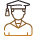#### 250M+

Students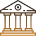#### 30,000+

Colleges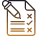#### 500+

Exams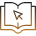E-Books